# TO CONSTRUCT THE REQUIRED ANGLE

## Constructions of Class 9

(a) To Construct the Required Angle of 60°:

STEPS:

(i)Draw a line BC of any suitable length.

(ii)With B as centre and any suitable radius, draw an arc which cuts BC at point D.

(iii) With D as centre and radius same, as taken in step (ii), draw one more arc which cuts previous arc at point E.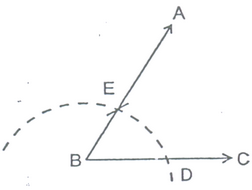(iv) Join BE and produce upto any point A.

Then, ∠ABC = 60o

(b)To Construct an Angle of 120°:

STEPS

(i)Draw a line BC of any suitable length.

(ii)Taking B as centre and with any suitable radius, draw an arc which cuts BC at point D.

(iii)Taking D as centre, draw an arc of the same radius, as taken in step (ii), which cuts the first arc at point E.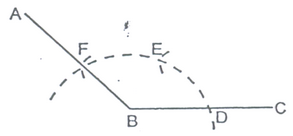(iv)Taking E as centre and radius same, as taken in step (ii), draw one more arc which cuts the first arc at point F.

(v) Join BF and produce upto any suitable point A.

Then, ∠ABC = 120o

(c) To Construct and Angle of  30°:

STEPS :

(i) Construct angle ABC = 60o by compass.

(ii) Draw BD, the bisector of angle ABC.

The, ∠DBC = 30o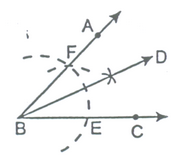(d) To Construct an Angle of 90°: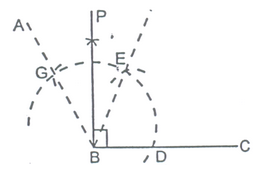STEPS:

(i) Construct angle ABC = 120o by using compass.

(ii) Draw PB, the bisector of angle EBG.

Then, ∠PBC = 90o

Alternative Method:

(i) Draw a line segment BC of any suitable length.

(ii) Produce CB upto a arbitrary point O.

(iii) Taking B as centre, draw as arc which cuts OC at points D and E.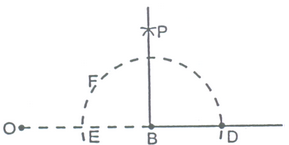(iv) Taking D and E as centres and with equal radii draw arcs with cut each other at point P.

(The radii in this step must be of length more than half of DE.)

(v)     Join BP and produce.

Then, ∠PBC = 90o

(d) To Construct an Angle of 45o

STEPS:

(i) Draw ∠PBC = 90o

(ii) Draw AB which bisects angle PBC,

Then, ∠ABC = 45o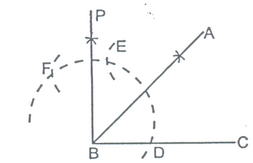Alternative Method:

STEPS: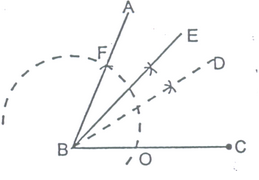(i) Construct ∠ABC = 60o

(ii) Draw BD, the bisector of angle ABC.

(iii) Draw BE, the bisector of angle ABD.

Then, ∠EBC = 45o

(e) To Construct an Angle of 105o:

STEPS: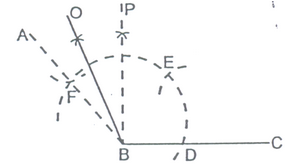(i) Construct ∠ABC = 120o and ∠PBC = 90o

(ii) Draw BO, the bisector of ∠ABP.

Then, ∠OBC = 105o

(f) To Construct an Angle of 150o.

STEPS:

(i)     Draw line segment BC of any suitable length. Produce CB upto any point O.

(ii)     With B as centre, draw an arc (with any suitable radius) which buts OC at points D and E.

(iii) With D as centre, draw an arc of the same radius, as taken in step 2, which cuts the first arc at point F.

(iv) With F as centre, draw one more arc of the same radius, staken in step 2, which cuts the first arc at point G.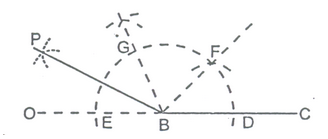(v) Draw PB, the bisector of angle EBG.

Now ∠FBD = ∠GBF = ∠EBG = 60o

Then, ∠PBC = 150o

(g) To Construct an Angle of 135o.

STEPS :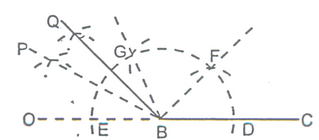(i) Construct ∠PBC = 150o and ∠GBC = 120o

(ii) Construct BQ, the bisector of angle PBG.

Then, ∠QBC = 135o